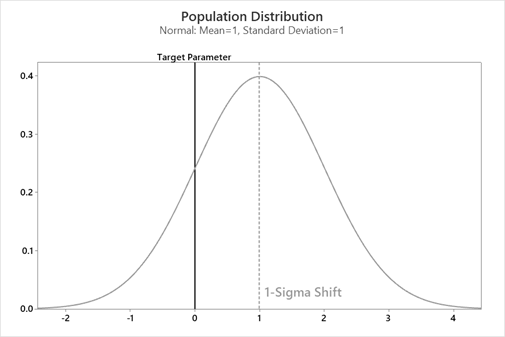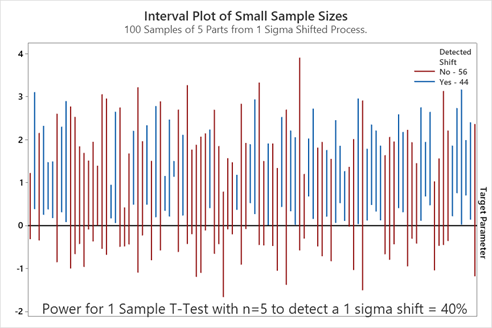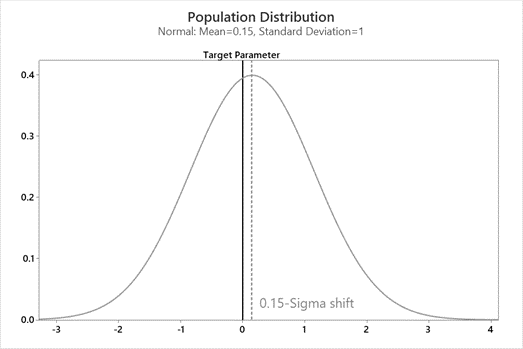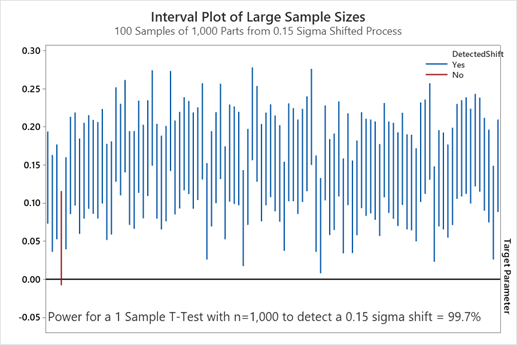The most practical reason for statistical analysis is the fact that a subset of data is collected and not the entire population. The flexibility of collecting sampled data saves money and time! This flexibility comes with a cost in errors in our decisions.

• Type I Error – False Positive – Producer’s Risk
• Type II Error – False Negative – Consumer’s Risk

These errors have different implications for analyst and can be exaggerated depending on the sample size used in the analysis. This blog post will cover these errors and how sample sizes can impact your conclusion.

## Power and Sample Size

People want to know the one number they should always use for a sample! They often will cite a sample size of 30 because someone at their company came across the Central Limit Theorem, but that number is not always correct.

In statistics, we use the concept of power to determine the probability of the sample size to detect a practical difference. The goal is to keep power high, a good target value of power is between 80% to 90%. Power can be considered the true positive rate of a test or think of it as a fire alarm that can detect a fire.

In Minitab Statistical Software, use the Stat > Power and Sample Size menu for the specific test you are implementing to make sure your sample size is correct.

## Type II Error and Not Enough Data

A Type II Error is the error of missing a critical difference. This is considered the false negative rate, the consumer’s risk, or think of this as a fire alarm that cannot detect a fire. You should be interested in the potential of a false negative if your p-value is greater than 0.05.

Type II error is considered the more egregious errors to make. In R+D, this could be a missed chance to optimize or improve a process. In manufacturing, this could be sending a bad part to a customer.

In this simulation, a moderate shift of a 1-sigma unit difference was generated off target. Below is the distribution of the 1-sigma shifted process and the target value.In this example, 100 samples of size 5 were pulled from the population to determine if they can detect the 1-sigma shift. In this example the power to detect a 1-sigma shift with a sample of size 5 is 40%. That means out of the 100 samples we have we should expect about 40 to detect the difference. In the simulation below, 44 of the samples were able to detect the shift since they did not capture the target.But this small sample size gives us a 60% chance of not detecting the shift in the process. We had less than a coin’s flip chance of determining the process is bad. With those low odds we might not realize the process needs fixing. And, in the real world, you would only do this once not 100 times.

With small sample sizes, the increase in false negative rates can give a sense of complacency and lead to no process improvement. To counteract the high potential of a false negative with small sample sizes, it is best to continuously monitor these processes using control charts or try to increase the sample size.

## Type I Error and Too Much Data

The Type I error is the error of detecting a negligible difference. This error is considered the false positive rate, the producer’s risk or you can think of it as a fire alarm that is going off when there is no fire. You should be considered of a potential false positive if the p-value is less than 0.05.

Below is an example where the process has shifted by 0.15-sigma units, this is considered a small and negligible difference and in this example no process improvements need to be carried out.From this 0.15-Sigma shifted process, 100 samples of 1,000 units were randomly sampled. Each of the 100 samples were then tested to see if they are off target. In this example, the test has a power of 99.7% in detecting the small shift of 0.15-sigma units Below is a graph of the 100 samples’ 95% confidence intervals. Of the 100 intervals, 99 do not capture zero indicating the process is off target.If the analyst is only looking at the p-value they might start implementing changes or “improvements." But if the analyst can see that the shift is only 0.15-sigma units then they would realize this is practically a false-alarm.

But would that small of a shift be necessary to invest resources to re-center the process to the target? The answer to that question is dependent on the cost, the specification limits of the product or the criticalness of the response variable.

To do robust analyses with large sample sizes users should consider machine learning techniques, like CART Classification Trees and CART Regression Trees or the users should compare the data in relation to specification limits or other pre-defined limits.

## Too much or not enough: Sample sizes and Statistical Analysis

Statistics make it cheaper and easier to make accurate business decisions, but these decisions can be drastically affected by the sample size used in the analysis. If there is not enough data, a false negative could be highly likely. If there is too much data, a false positive could be a problem. Strong historical knowledge, good data collection processes and a robust understanding of statistical analysis will make any decision powerful.